Examples

Chapter 5 Class 11 Linear Inequalities
Serial order wise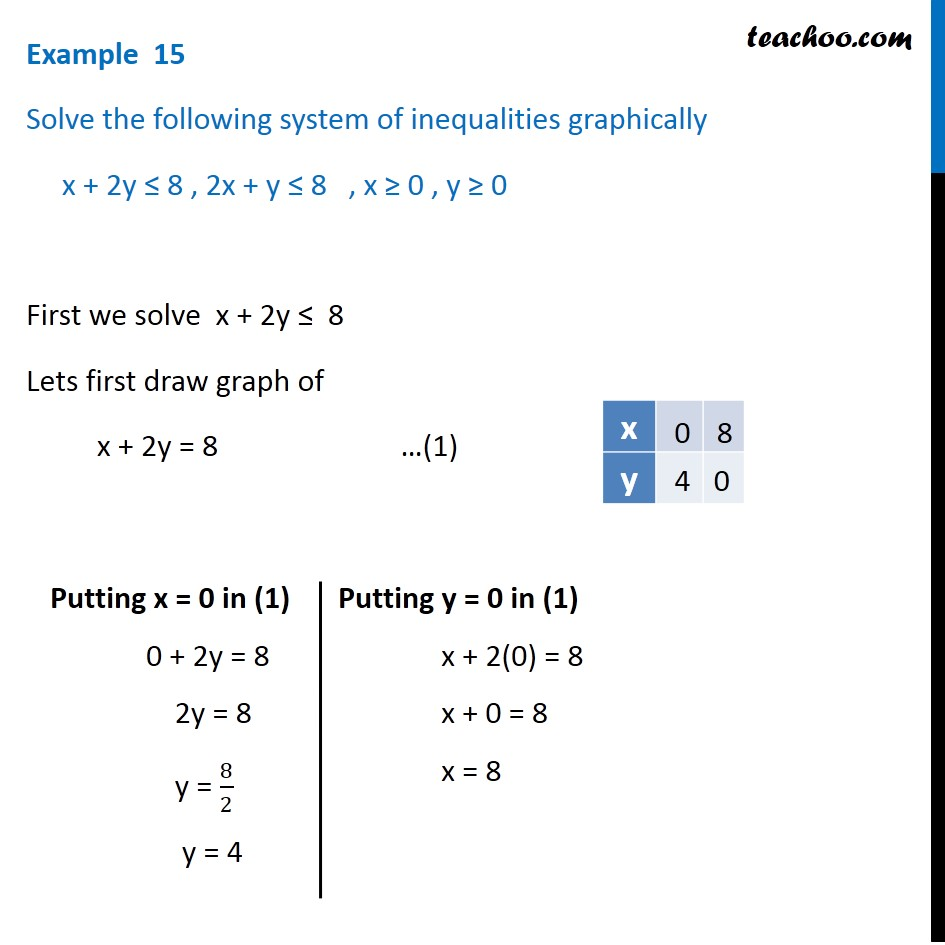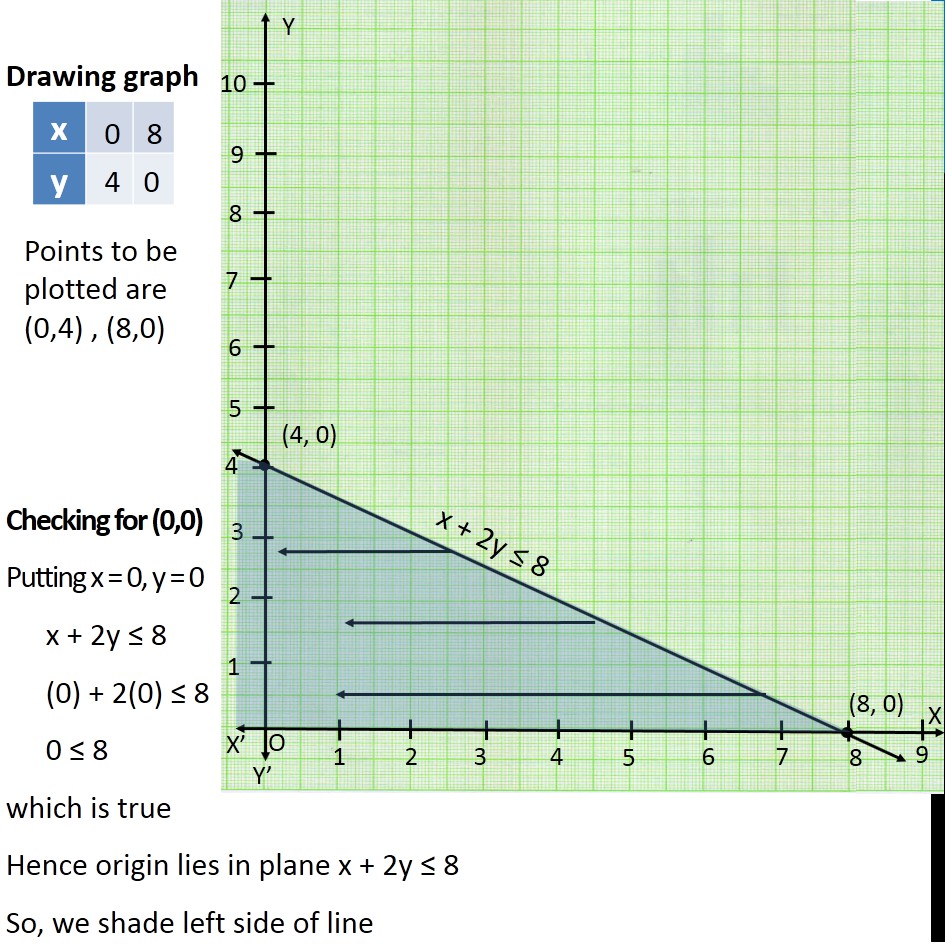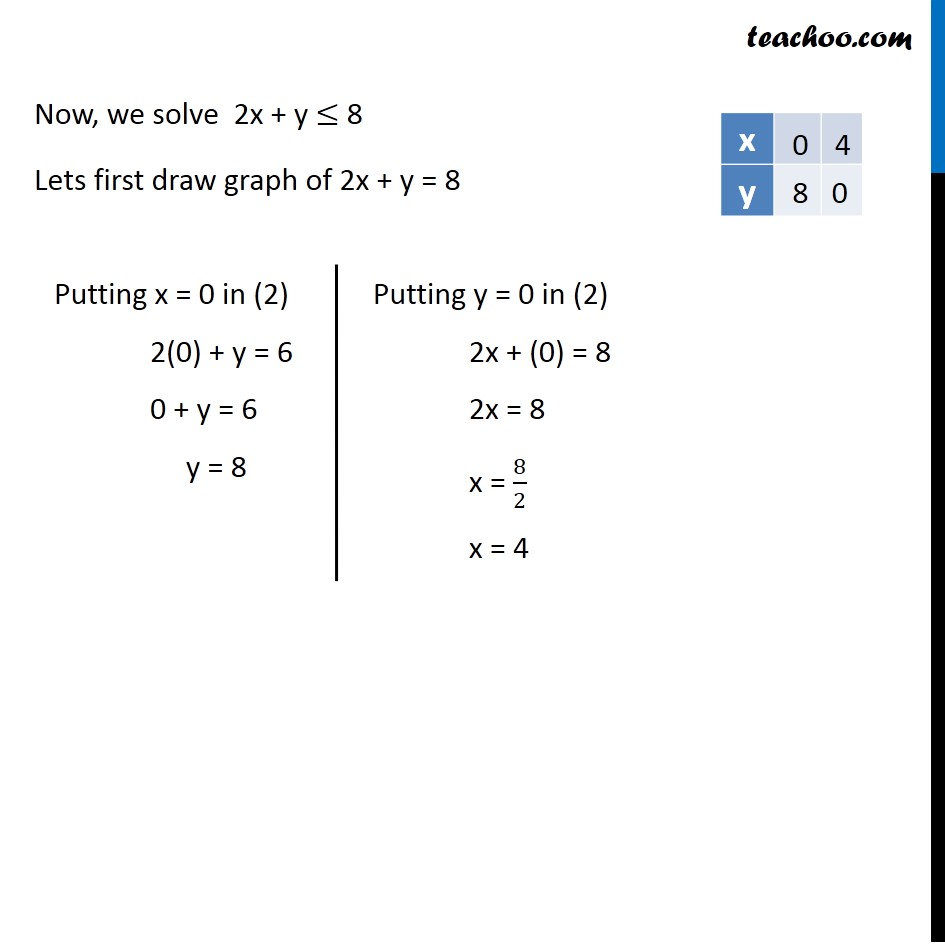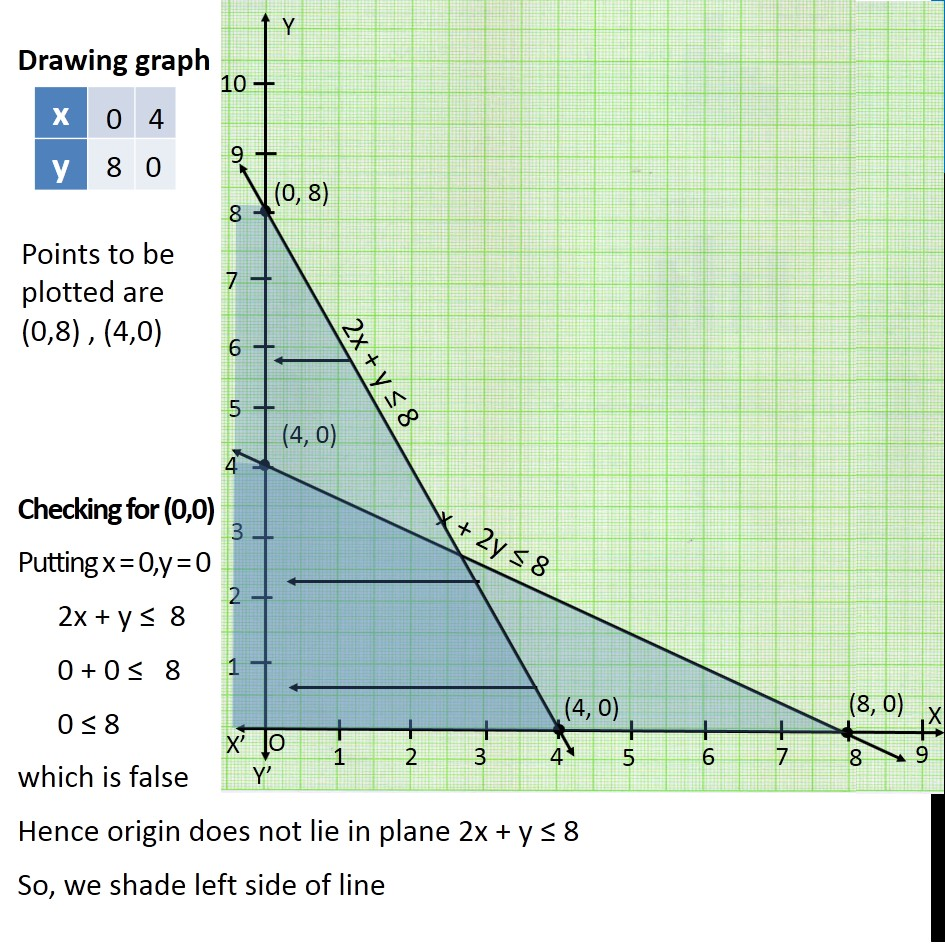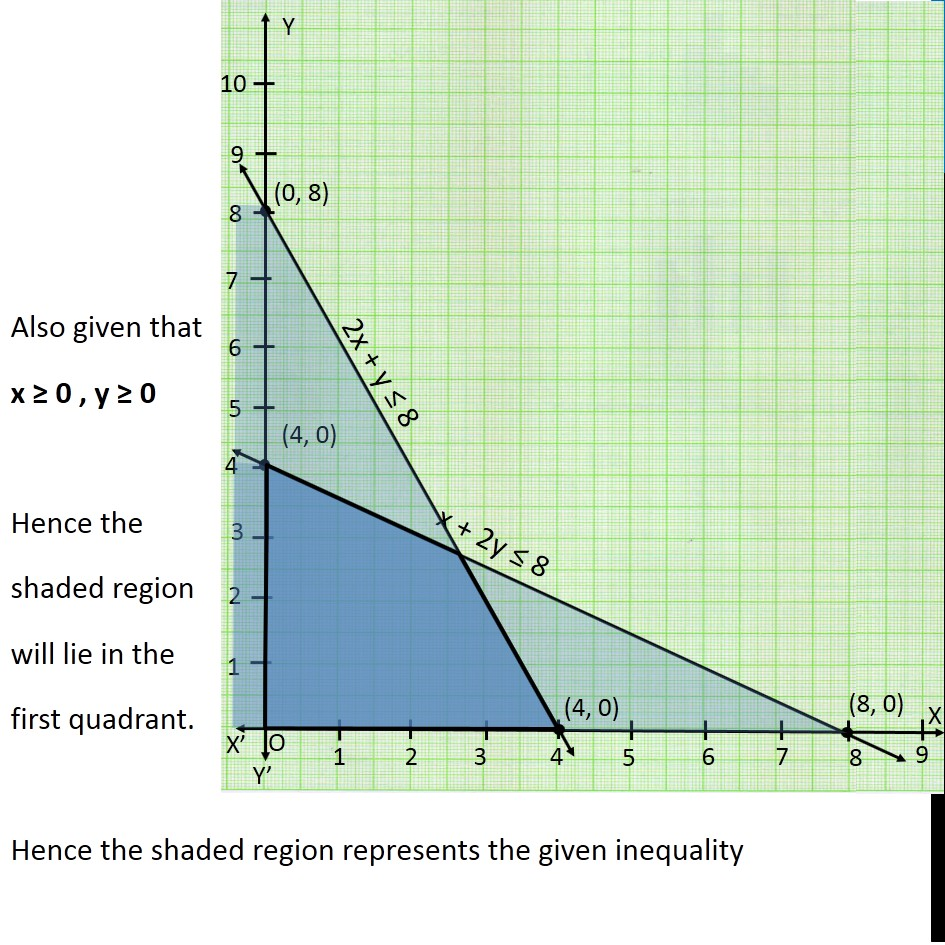Learn in your speed, with individual attention - Teachoo Maths 1-on-1 Class

### Transcript

Question 7 Solve the following system of inequalities graphically x + 2y ≤ 8 , 2x + y ≤ 8 , x ≥ 0 , y ≥ 0 First we solve x + 2y ≤ 8 Lets first draw graph of x + 2y = 8 Putting x = 0 in (1) 0 + 2y = 8 2y = 8 y = 8/2 y = 4 Putting y = 0 in (1) x + 2(0) = 8 x + 0 = 8 x = 8 Points to be plotted are (0,4) , (8,0) Drawing graph Checking for (0,0) Putting x = 0, y = 0 x + 2y ≤ 8 (0) + 2(0) ≤ 8 0 ≤ 8 which is true Hence origin lies in plane x + 2y ≤ 8 So, we shade left side of line Now, we solve 2x + y ≤ 8 Lets first draw graph of 2x + y = 8 Putting x = 0 in (2) 2(0) + y = 6 0 + y = 6 y = 8 Putting y = 0 in (2) 2x + (0) = 8 2x = 8 x = 8/2 x = 4 Points to be plotted are (0,8) , (4,0) Drawing graph Checking for (0,0) Putting x = 0,y = 0 2x + y ≤ 8 0 + 0 ≤ 8 0 ≤ 8 which is false Hence origin does not lie in plane 2x + y ≤ 8 So, we shade left side of line Also given that x ≥ 0 , y ≥ 0 Hence the shaded region will lie in the first quadrant. Hence the shaded region represents the given inequality